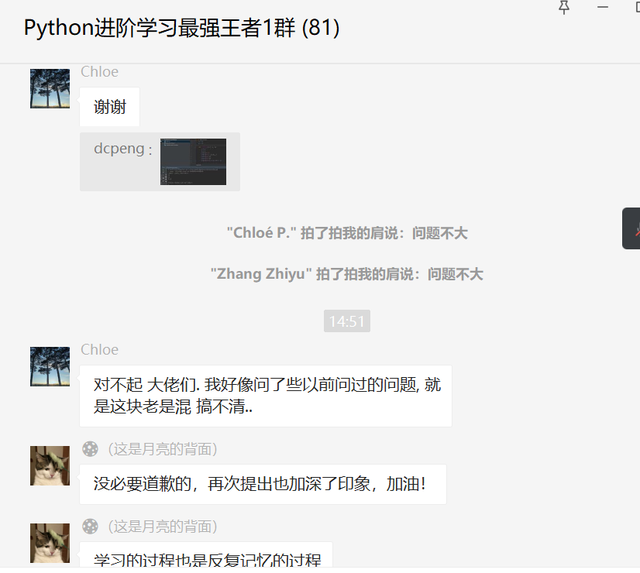# 一、前言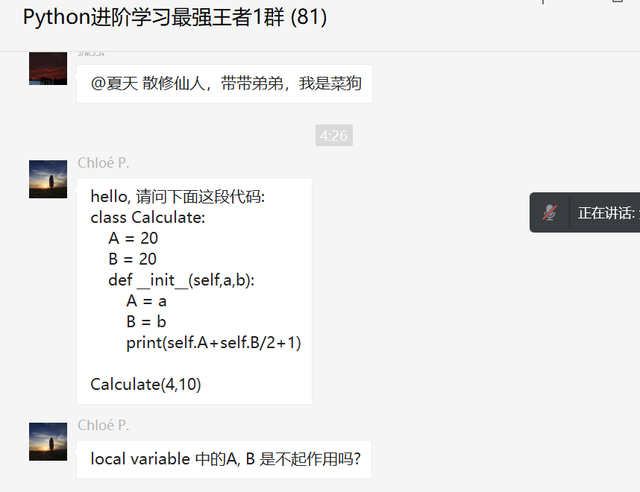# 二、解决过程

Generally speaking, instance variables are for data unique to each instance and class variables are for attributes and methods shared by all instances of the class.

``````class Calculate:
A = 20
B = 20
def __init__(self,a,b):
A = a
B = b
print(self.A+self.B/2+1)

Calculate(4,10)
``````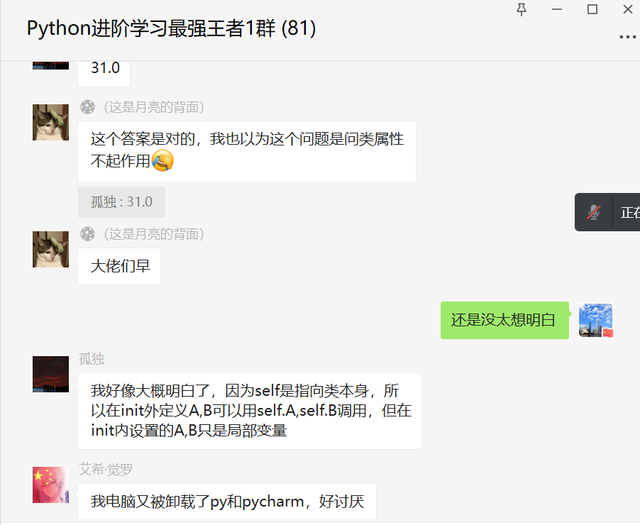``````class Calculate:
A = 20
B = 20
def __init__(self,a,b):
self.A = a
self.B = b
print(self.A+self.B/2+1)

Calculate(4,10)
``````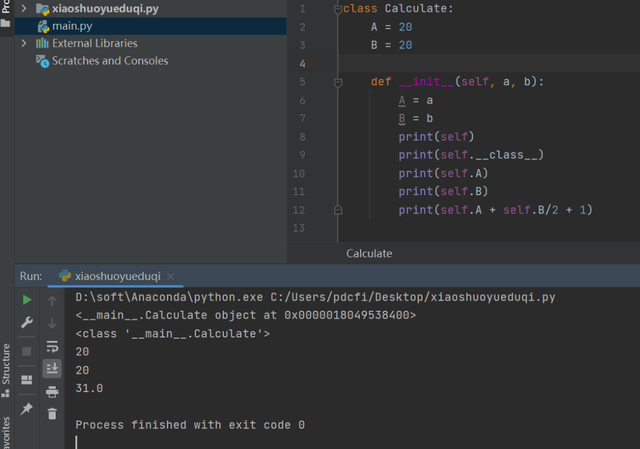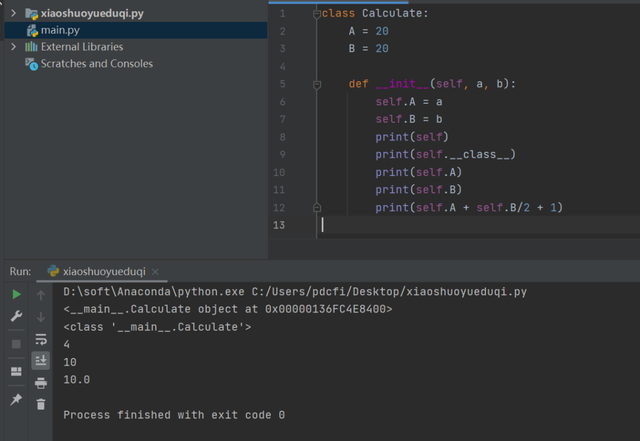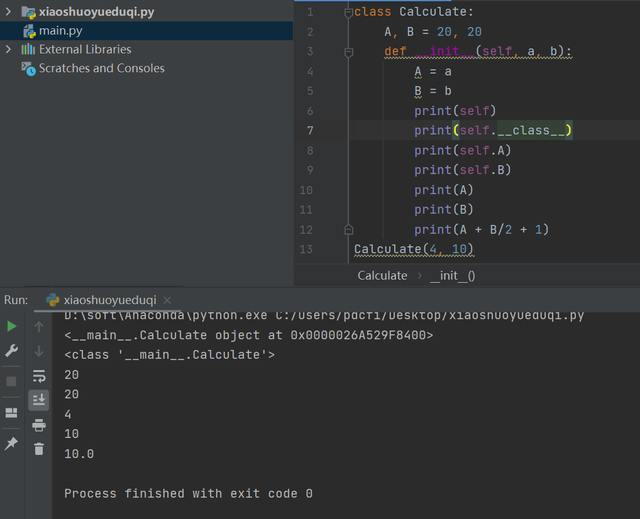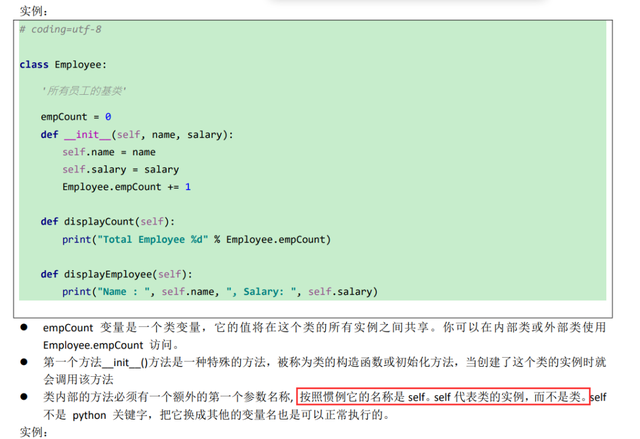# 三、总结